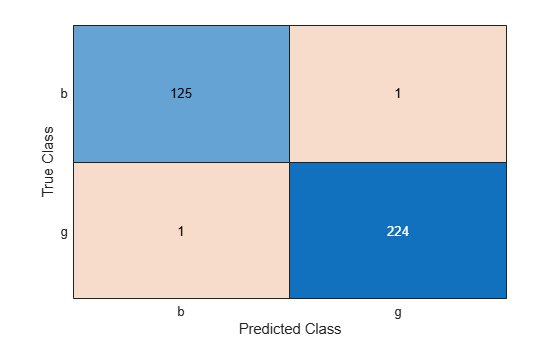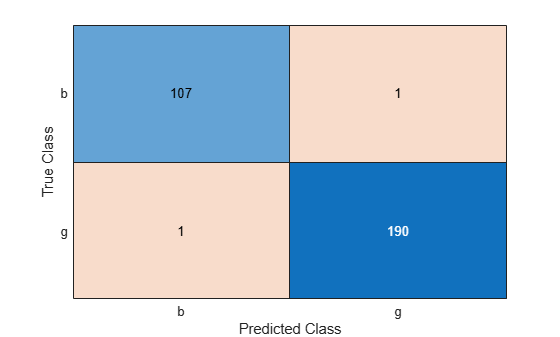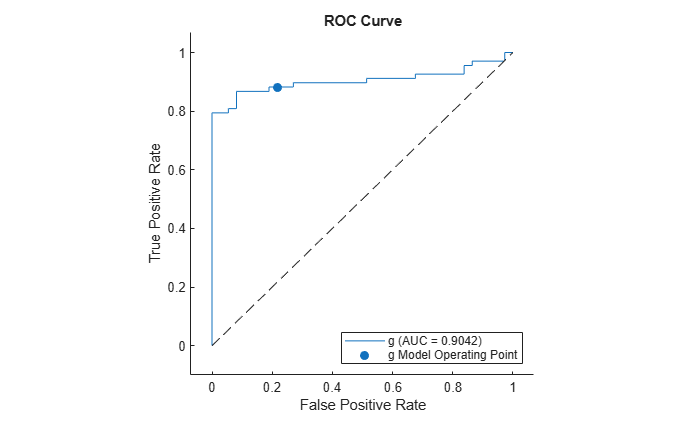Documentation

# predict

Predict labels for Gaussian kernel classification model

## Syntax

``Label = predict(Mdl,X)``
``[Label,Score] = predict(Mdl,X)``

## Description

example

````Label = predict(Mdl,X)` returns predicted class labels for each observation in the predictor data `X` based on the binary Gaussian kernel classification model `Mdl`.```

example

````[Label,Score] = predict(Mdl,X)` also returns classification scores for both classes.```

## Examples

collapse all

Predict the training set labels using a binary kernel classification model, and display the confusion matrix for the resulting classification.

Load the `ionosphere` data set. This data set has 34 predictors and 351 binary responses for radar returns, either bad (`'b'`) or good (`'g'`).

`load ionosphere`

Train a binary kernel classification model that identifies whether the radar return is bad (`'b'`) or good (`'g'`).

```rng('default') % For reproducibility Mdl = fitckernel(X,Y);```

`Mdl` is a `ClassificationKernel` model.

Predict the training set, or resubstitution, labels.

`label = predict(Mdl,X); `

Construct a confusion matrix.

`ConfusionTrain = confusionchart(Y,label);`The model misclassifies one radar return for each class.

Predict the test set labels using a binary kernel classification model, and display the confusion matrix for the resulting classification.

Load the `ionosphere` data set. This data set has 34 predictors and 351 binary responses for radar returns, either bad (`'b'`) or good (`'g'`).

`load ionosphere`

Partition the data set into training and test sets. Specify a 15% holdout sample for the test set.

```rng('default') % For reproducibility Partition = cvpartition(Y,'Holdout',0.15); trainingInds = training(Partition); % Indices for the training set testInds = test(Partition); % Indices for the test set```

Train a binary kernel classification model using the training set. A good practice is to define the class order.

`Mdl = fitckernel(X(trainingInds,:),Y(trainingInds),'ClassNames',{'b','g'}); `

Predict the training-set labels and the test set labels.

```labelTrain = predict(Mdl,X(trainingInds,:)); labelTest = predict(Mdl,X(testInds,:));```

Construct a confusion matrix for the training set.

`ConfusionTrain = confusionchart(Y(trainingInds),labelTrain);`The model misclassifies only one radar return for each class.

Construct a confusion matrix for the test set.

`ConfusionTest = confusionchart(Y(testInds),labelTest);`Estimate posterior class probabilities for a test set, and determine the quality of the model by plotting a receiver operating characteristic (ROC) curve. Kernel classification models return posterior probabilities for logistic regression learners only.

Load the `ionosphere` data set. This data set has 34 predictors and 351 binary responses for radar returns, either bad (`'b'`) or good (`'g'`).

`load ionosphere`

Partition the data set into training and test sets. Specify a 30% holdout sample for the test set.

```rng('default') % For reproducibility Partition = cvpartition(Y,'Holdout',0.30); trainingInds = training(Partition); % Indices for the training set testInds = test(Partition); % Indices for the test set```

Train a binary kernel classification model. Fit logistic regression learners.

```Mdl = fitckernel(X(trainingInds,:),Y(trainingInds), ... 'ClassNames',{'b','g'},'Learner','logistic');```

Predict the posterior class probabilities for the test set.

`[~,posterior] = predict(Mdl,X(testInds,:));`

Because `Mdl` has one regularization strength, the output `posterior` is a matrix with two columns and rows equal to the number of test-set observations. Column `i` contains posterior probabilities of `Mdl.ClassNames(i)` given a particular observation.

Obtain false and true positive rates, and estimate the area under the curve (AUC). Specify that the second class is the positive class.

```[fpr,tpr,~,auc] = perfcurve(Y(testInds),posterior(:,2),Mdl.ClassNames(2)); auc```
```auc = 0.9042 ```

The AUC is close to `1`, which indicates that the model predicts labels well.

Plot an ROC curve.

```figure; plot(fpr,tpr) h = gca; h.XLim(1) = -0.1; h.YLim(2) = 1.1; xlabel('False positive rate') ylabel('True positive rate') title('ROC Curve')```## Input Arguments

collapse all

Binary kernel classification model, specified as a `ClassificationKernel` model object. You can create a `ClassificationKernel` model object using `fitckernel`.

Predictor data, specified as an n-by-p numeric matrix, where n is the number of observations and p is the number of predictors used to train `Mdl`.

Data Types: `single` | `double`

## Output Arguments

collapse all

Predicted class labels, returned as a categorical or character array, logical or numeric matrix, or cell array of character vectors.

`Label` has n rows, where n is the number of observations in `X`, and has the same data type as the observed class labels (`Y`) used to train `Mdl`. (The software treats string arrays as cell arrays of character vectors.)

`predict` classifies observations into the class yielding the highest score.

Classification scores, returned as an n-by-2 numeric array, where n is the number of observations in `X`. `Score(i,j)` is the score for classifying observation `i` into class `j`. `Mdl.ClassNames` stores the order of the classes.

If `Mdl.Learner` is `'logistic'`, then classification scores are posterior probabilities.

collapse all

### Classification Score

For kernel classification models, the raw classification score for classifying the observation x, a row vector, into the positive class is defined by

`$f\left(x\right)=T\left(x\right)\beta +b.$`

• $T\left(·\right)$ is a transformation of an observation for feature expansion.

• β is the estimated column vector of coefficients.

• b is the estimated scalar bias.

The raw classification score for classifying x into the negative class is f(x). The software classifies observations into the class that yields a positive score.

If the kernel classification model consists of logistic regression learners, then the software applies the `'logit'` score transformation to the raw classification scores (see `ScoreTransform`).